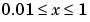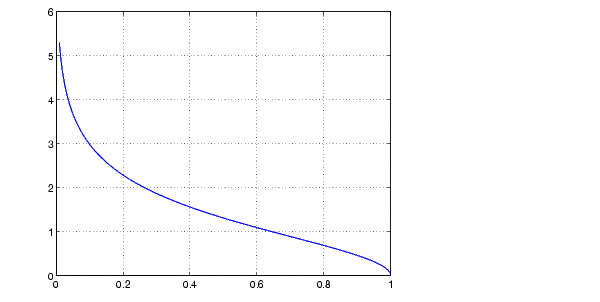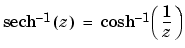MATLAB Function Referenceasech

Inverse hyperbolic secant

Syntax

• ```Y = asech(X)
```

Description

```Y = asech(X) ``` returns the inverse hyperbolic secant for each element of `X`.

The `asech` function operates element-wise on arrays. The function's domains and ranges include complex values. All angles are in radians.

Examples

Graph the inverse hyperbolic secant over the domain.

• ``````x = 0.01:0.001:1;
``````plot(x,asech(x)), grid on
``````

Definition

The hyperbolic inverse secant can be defined as

•Algorithm

`asech` uses FDLIBM, which was developed at SunSoft, a Sun Microsystems, Inc. business, by Kwok C. Ng, and others. For information about FDLIBM, see http://www.netlib.org.

See Also

`asec`, `sech`

© 1994-2005 The MathWorks, Inc.